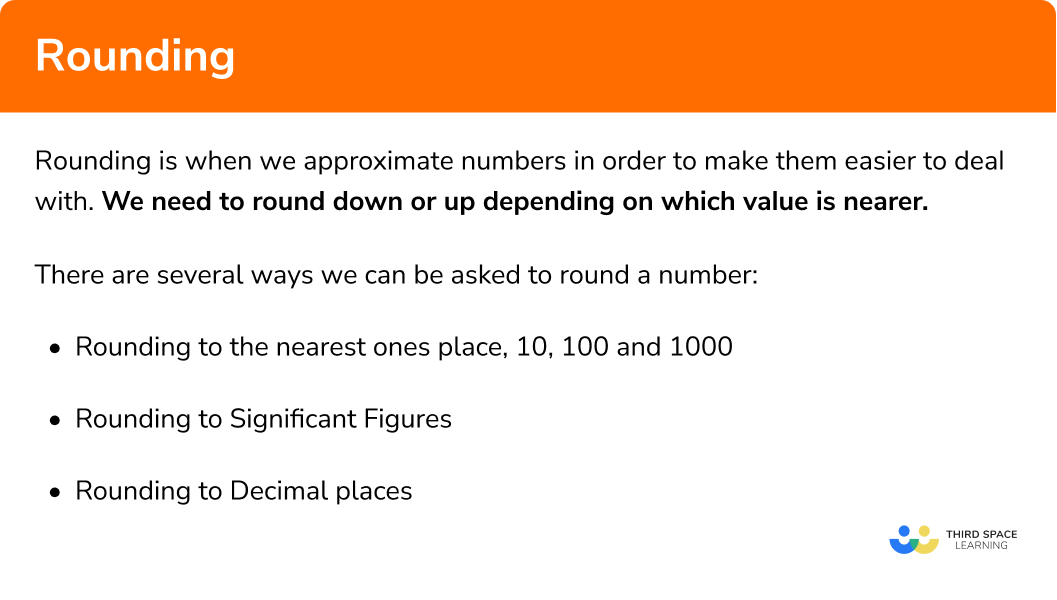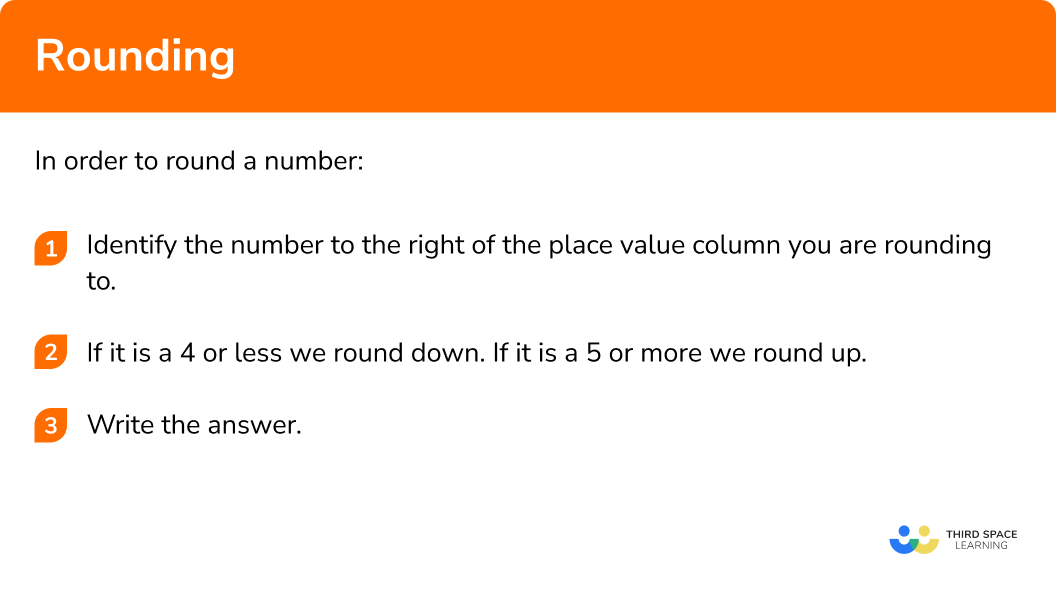GCSE Maths Number

Rounding Numbers

# Rounding Numbers

Here we will learn about rounding including how to round a number to the nearest 10, 100 or 1000, how to round a number to a given number of decimal places and how to round a number to a given number of significant figures. We will also learn about truncation and how we can also use rounded numbers to estimate the solution to a calculation.

There are also rounding worksheets based on Edexcel, AQA and OCR exam questions, along with further guidance on where to go next if you’re still stuck.

## What is rounding?

Rounding is when we approximate numbers in order to make them easier to deal with. We need to round down or up depending on which value is nearer.

There are several ways we can be asked to round a number.

E.g.

1478.27 can be rounded in several ways

• Rounding to the nearest ones place, 10, 100 and 1000
1478.47 is 1478 rounded to the nearest ones place
1478.47 is 1480 rounded to the nearest 10
1478.47 is 1500 rounded to the nearest 100
1478.47 is 1000 rounded to the nearest 1000
• Rounding to significant figures
1478.47 is 1000 rounded to 1 significant figure
1478.47 is 1500 rounded to 2 significant figures
1478.47 is 1480 rounded to 3 significant figures
• Rounding to decimal places
1478.2735 is 1478.3 rounded to 1 decimal place (the nearest tenth)
1478.2735 is 1478.27 rounded to 2 decimal places (the nearest hundredth)
1478.2735 is 1478.274 rounded to 3 decimal places (the nearest thousandth)

Truncating a number and estimation are also linked with rounding:

• Truncation is cutting a number off at a certain value with no need to round up.
• Estimation is using the approximated numbers for a calculation; we do not use the exact numbers.

### What is rounding?## How to round numbers

In order to round a number:

1. Identify the number to the right of the place value column you are rounding to
2. If it is a 4 or less we round down, if it is a 5 or more we round up

### Explain how to round a number in 3 steps## Rounding numbers examples

### Example 1: rounding to the nearest 10, 100 and 1000

Round 382 to the nearest 10.

1. Identify the number to the right of the place value column you are rounding to

We are rounding to the nearest 10 so we identify the number to the right of the 8 in the tens column.
This is a 2.

2 If it is a 4 or less we round down, if it is a 5 or more we round up

It is a 2 is 4 or less so we round down to 380.

382 is 380 rounded to the nearest 10

Step-by-step guide: Rounding to the nearest 10, 100 and 1000

### Example 2: rounding to decimal places

Round 14.76 to 1 decimal place (to 1 dp).

We are rounding to 1 decimal place so we identify number to the right of the 7 in the first decimal place.
This is a 6

6 is more than 5 so we round up to 14.8

14.76 is 14.8 rounded to 1 decimal place.

Step-by-step guide: Decimal places

### Example 3: rounding to decimal places

Round 25.673 to the nearest whole number.

We are rounding to the nearest whole number so we identify the number to the right of the 5 in the ones column.
This is a 6.

6 is more than 5 so we round up to 26

25.673 is 26 rounded to the nearest whole number.

Step-by-step guide: Rounding decimals

### Example 4: rounding to significant figures

Round 357 to 1 significant figure.

We are rounding to 1 significant figure so we identify the number to the right of 3 in the hundreds column as this is the first significant figure.
This is a 5

As the digit is a 5 we round up to 400

357 is 400 rounded to 1 significant figure.

Step-by-step guide: Significant figures

### Example 5: rounding to significant figures

Round 7.5823 to 2 significant figures (to 2 sf).

We are rounding to 2 significant figures so we identify the number to the right of the 5 in the tenths column as this is the second significant figure.
This is an 8.

8 is more that 5 so we round up to 7.6.

7.5823 is 7.6 rounded to 2 significant figures.

Step-by-step guide: Upper and lower bounds

### Example 6: truncation

Truncate 94.5871 to 2 decimal places (to 2 dp).

We are truncating to 2 decimal places so we identify the number in the second decimal place.
This is an 8.

As we have been asked to truncate the number we can miss off all of the digits after the second decimal place.

94.5871 is 94.58 truncated to 2 decimal places.

Step-by-step guide: Truncation

### Example 7: estimation

Estimate the following calculation:

287.1 × 14.7

As we are estimating we need to round each number to 1 significant figure.

In 287.1 we identify the number to the right of the first significant figure.
This is an 8.

In 14.7 we identify the number to the right of the first significant figure.
This is a 4.

8 is more than 5 so we round 287.1 up to 300.

4 is less than 5 so we round 14.7 down to 10.

The estimated calculation is 300 × 10 = 3000

The estimated answer is is 3000

Step-by-step guide: Estimation

### Common misconceptions

• Remember to put zero in as a place holder before the decimal point

E.g.
When we round 2769.1 to the nearest 100 the answer will be 2700 because we need zeros as place holders in the tens and units columns.

2739.1 ≈ 2700

NOT 27

• Sometimes we need a zero in as a place holder with decimal numbers

E.g.
When we round 3.4973 to 2 decimal places the answer will be 3.50 because we need the zero in the hundredths column to show the two decimal places.

3.4973 ≈ 3.50

• Estimation of numbers within square roots

If your estimation involves a square root symbol, round those numbers to the nearest square number.

E.g.

$13.45 + \sqrt{23.6} \approx10 + \sqrt{25} = 10 +5 = 15$

### Rounding practice questions

1. Round 193 to the nearest 100 .

10020012We are rounding to the nearest 100 . Identifying the 9 in the tens column indicates we need to round up.

2. Round 13.782 to 2 decimal places.

13.7913.7813.78013.00We are rounding to 2 decimal places. The 2 in the thousandths column indicates that we should round down.

3. Round 5692 to 2 significant figures.

5600600057005690We are rounding to 2 significant figures. The 9 in the tens column indicates we round up. We need to have zeros in the tens and ones columns as place holders.

4. Round 0.0365 to 2 significant figures.

0.030.30.0360.037We are rounding to 2 significant figures. The second significant figure is 6 .

The 5 in the ten thousandths column indicates we round the 6 up.

5.  Truncate 78 to the nearest 10 .

7870807We locate the digit in the tens column and truncate to this degree of accuracy, replacing the digit in the ones column with a zero.

6.  Estimate   341 × 27 .

9207900068009200By first rounding each number to one significant figure, the calculation becomes

300\times30 = 9000

### Rounding GCSE Questions

1. Write 78 348 correct to the nearest 1000 .

(1 Mark)

78 000

(1)

2. (a)  Write 8400 correct to one significant figure.

(b) Write 3.51 correct to one significant figure.

(2 Marks)

(a)

8000

(1)

(b)

4

(1)

3. Work out an estimate for

\frac{572\times213}{36} .

(2 Marks)

For rounding one original number to 1 significant figure 600 or 200 or 40

\frac{600\times200}{40}

(1)

For the calculation involving all three correctly rounded numbers.

(1)

= 3000

(1)

4. (a)  Use your calculator to work out

\frac{65.4+49.3}{23.4-15.8}

Write down all the figures on your display.

(2 Marks)

(a) 15.092105…

(1)

(b)   15.1 (1.d.p)

(1)

## Learning checklist

You have now learned how to:

• Round numbers to a number of decimal places
• Round numbers to a number of significant figures
• Use approximation through rounding to estimate answers

## Still stuck?

Prepare your KS4 students for maths GCSEs success with Third Space Learning. Weekly online one to one GCSE maths revision lessons delivered by expert maths tutors.

Find out more about our GCSE maths tuition programme.